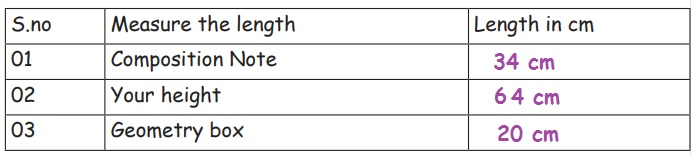Home | | Maths 5th Std | Length

# Length

Able to apply the four operation in solving problems involving length.

UNIT 4

MeasurementsLength

a. Able to apply the four operation in solving problems involving length.

Introduction

In day to day life, we are measuring many small things by standard and non standard units. But how can we measure the distance.The metric units are used to measure the length and distance.

I am measurika. I would like to measure:1. What is the length of the table?

2. How tall am I?

3. How far is my school from my home?

How do I measure it? What kind of measurements are they?

Ponni explained her that how long things are, how tall they are, or how far apart they might be. These are all the examples of length.

Measaurika, you should know the following measurements:

a. Millimeter (mm): Millimeters is the smaller unit of length. A millimeter is about the thickness of a plastic id card (or Debit/ credit card). Or about the thickness of 10 sheets of paper on top of each other.

This is a very small measurement!b. Centimeter: 10 millimeters is equal to 1 centimeter

1 centimeter = 10 millimeters

A fingernail is about one centimeter wide.

We can use millimeters or centimeters to measure how tall we are, or how wide a table is. But to measure the length of football field, it is better to use meters.Could you please mark 4mm in red colour and 10mm in Green colour?Could you mark 1cm and 3mm (1.3cm) ?

10 milli meter = 1 centi meter

10 centi meter = 1 deci meter

10 deci meter = 1 meter

10 meter = 1 deca meter

10 deca meter = 1 hecta meter

10 hecta meter = 1 killo meterc. Meter

A meter is equal to 100 centimeters

The length of this guitar is about 1 meter

Meters are used to measure the length of a house, or the size of a play ground.

One meter is approximately the length from your shoulder to your fingertips. A meter is also approximately the distance of one large step or jump.

A Measuring tape has centimeter and meter units marked on it. Measuring tapes are useful for measuring lengths of cloth, or large household objects like furniture and rooms.d. Kilometer

When you need to get from one place to another, you can measure the distance using kilometers. A kilometer is equal to 1,000 meters.

The distance from one city to another or how far a plane travels is measured using kilometers.

We can ride a Two wheeler/ Four wheeler to go from one place to the other. The distance travelled is measured using the speedometer.

1Km = 1000mTags : Measurements | Term 1 Chapter 4 | 5th Maths , 5th Maths : Term 1 Unit 4 : Measurements
Study Material, Lecturing Notes, Assignment, Reference, Wiki description explanation, brief detail
5th Maths : Term 1 Unit 4 : Measurements : Length | Measurements | Term 1 Chapter 4 | 5th Maths